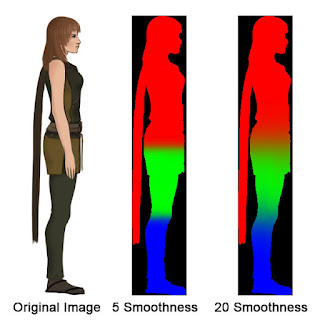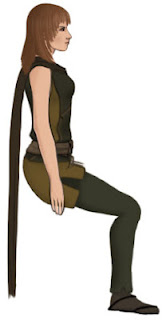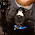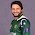## Thursday, July 26, 2012

### Bones and Automatic Vertex Weights

In my last post, I explained how VIDE vectorizes images which allows the image to be deformed by changing the vertex positions. However, manually changing the vertex positions is very tedious, and not artist friendly. A lot of animations, especially character animations, usually involve rotating limbs around joints. Bones allow an animator to do this easily by drawing bones and joints, and then deform large portions of the vectorized image by simply rotating the bone. For this to work, every vertex must be assigned a weight for every bone so that when a bone rotates, the right vertices will rotate with it. The simplest way to assign these weights is based on distance to the bone – the closer the bone is to the vertex, the higher the weight, so that the leg bone will affect vertices near the leg, and not vertices in the head. However, euclidean (straight line) distance will allow bone weights to “bleed” across gaps. This is easy to see when you have two legs next to each other in the image – a bone in one leg will affect the vertices in the other leg as the straight-line distance can cross the gap between the two legs. What we really need is geodesic distance, which is shortest distance within the space of the vectorized image. Since the distance is constrained to the space of the vectorized image, it cannot cross gaps or leave the image’s bounds, so the geodesic distance from the left leg to the right leg is the distance up the left leg and down the right leg (without crossing the gap between the two legs), solving the weight bleeding across gaps. A good analogy is that euclidean distance is how the crow flies, and geodesic distance is the way you have to walk.The weight from the lower leg bone, using the exponential rule with 10 smoothness and 100 smoothness. Note that the higher smoothness spreads the bone’s weight further than the lower smoothness. Also note that the cape has a low weight even though it is very close to the bone straight-line distance.
So now we have to compute the geodesic distance from every bone to every vertex. To solve this problem, I did something similar to Volumetric Heat Diffusion, which you can read about on wolfire’s blog: http://blog.wolfire.com/2009/11/volumetric-heat-diffusion-skinning/. They also have a good example of the weight bleeding effect. Their idea is simple: take a discretized version of the model (in 3D, they need to use a voxel-grid, but in 2D, we can simply use our alpha-thresholded image), and then spreads the weight of the bone throughout the entire model as if it were a heating element. This is done by setting each voxel’s heat to the average of its neighbors iteratively until convergence. One it converges, they can lookup the “heat” of the voxel at the location of each vertex and use that to compute the weight of that bone. Once converged, the “heat” is proportional to the geodesic distance (which our friend Laplace can confirm for us), but convergence can take a lot of iterations, with each iteration requiring a loop over all of the pixels in the voxel-grid or image. As you can imagine, this can be quite slow, especially without access to the parallel processing power of the graphics card. So, I thought: why not just compute the actual geodesic distance in one iteration? While not embarrassingly parallel like the above method, Dijkstra's algorithm does just that. Set the initial distance of all pixels in our image to infinity (or, if you use a 32-bit image like I did, an integer value which is sufficiently large). Then, set all of the pixels along the bone to 0, and add all of those pixels to a working queue. This can be done by treating the bone as a line, and using a line-rasterization algorithm to get all of the pixels in the image along that line. Now, until the working queue is empty, dequeue the next pixel, and for every neighboring pixel that is within our outline (using the same alpha-threshold as we did to generate the vectorized image) and is unvisited (meaning its distance is less than infinity), set that pixel’s distance to the current pixel’s distance plus one, and add it to the queue. Visually, this is quite simple, the distance along the bone is zero, the distance of pixels adjacent to the bone is one, and so on.The generated normalized weights for the body bone (red), the upper leg bone (green), and the lower leg bone (blue). Note how the higher smoothness has a smoother transition of weights at the joints.
For those of you who know Dijkstra's algorithm, my algorithm is not quite the same, it’s an optimization assuming that the distance from one pixel to any neighboring pixel is the same (which it is as we always add one to the distance). Also, for those of you who really like to analyze algorithms, you may notice that this means that the distance from one pixel to a diagonal pixel is 2, not v2. This means that we aren’t really getting the shortest distance within the outline, but the shortest manhattan distance within the outline. This can be fixed by following Dijkstra's algorithm without my optimization and including the diagonals as neighbors with a weight of v2, but this requires additional computation and updates of pixels, and does not make a significant difference in the assigned weights of the bones.The result of the above bone weights with the bones bent into a sitting position. The higher smoothness gives a smooth bend, but looks too smooth at the hip joint. The lower smoothness only bends a small part of the joint.
So, now that we have the distances computed, how do we actually assign the vertex weights? Obviously, the larger the distance, the less the weight, but how much less? The answer to that is: it depends! If the weight fades a lot with distance, then you get a hard, angular joint that is good for elbows. If the weight fades slowly with distance, then you get a soft, smooth joint that is good for backs and hair. I found that an exponential function tends to work well: e^(-distance/smoothness). This function is always one for zero distance, and drops off quickly with a low smoothness, and slowly with a high smoothness. Let the artists decide what smoothness is best. Don’t forget to normalize the vertex weights so that they add to one! Also, you do not need to store all of the bone weights per vertex - usually storing the four highest weighted bones is enough. Then, to transform the vertices, compute the transformation matrices for each bone, and then the transformed vertex position is the sum of the vertex position transformed by each bone’s matrix weighted by the bone’s weight. Obviously, if a bone’s weight is one, then the vertex is transformed by just that bone, and at the joints, it will smoothly interpolate between the transformations of the nearby bones.A sample showing the how layers will work in VIDE. The arm does not bend with the body in this example as it is in a different layer, and can rotate independently.
We now have a working system that can deform images based on bones. VIDE is done now right? Unfortunately, making this tool usable will require layers, animation tracks, and all sorts of UI stuff. But the point is that we can now animate and deform images! Who cares if anyone can use the program or not, right? All joking aside, look forward to more updates on VIDE, as well as updates on some of the game projects I’m currently working on.

1.Just wanted to say this post was extremely informative and helpful. I'm working on something similar so the technical talk really helped!

2.One question I had, I'm working on a mesh that is procedurally generated. Looks like this http://forum.unity3d.com/threads/185377-Procedural-mesh-skinning?p=1290503#post1290503

In your post you refer to pixels. So the algorithm runs on the pixels and calculates the distance that way, then the vertices look up the distance by matching their location to a pixel using the pixel grid? . This post never shows the underlying mesh so I was just curious.

I ask this because I'm working with Unity on mobile and doing anything with pixels is really slow so I am trying to avoid that.

Thanks!

3.I explain how I generate the mesh from the images in this post: http://david.fancyfishgames.com/2012/07/introduction-to-vide.html . Since I use an image as a base, I already have the pixels defined, but you could rasterize your mesh (at lower resolutions to save computation time - the lower the resolution, the more approximate the distance) and compute the distance on that. You could also attempt a different distance metric, but you really need geodesic distance and not euclidean or else you'll get bone bleeding (there are other algorithms to approximate geodesic distance, but none of them are cheap/easy). Running this on mobile will be slow (5-15 seconds per mesh), if you're generating a lot of characters you wont be able to do this real time. If a lot of the characters have the same general shape, you could compute the bones/weights once, and then use an alpha texture to change the appearance of different characters (reusing the bones).

Hope that helped! Let me know if you have more questions.

4.Thanks David! That was helpful. Ok just a few more questions I promise.

Here you say:

"take a discretized version of the model (in 3D, they need to use a voxel-grid, but in 2D, we can simply use our alpha-thresholded image)"

I'm having trouble understanding what the underlying purpose of this is, as opposed to running the algorithm on the raw verts themselves? Does it make it simpler/faster if everything is laid out on a grid and the distance between everything is 1?

I have a 1st version of Dijkstra's algorithm working. Many of the examples I found use it for path finding, with a source point and target point. Since we aren't trying to find a specific path, would it be safe to say we are trying to find the shortest path possible path from the bone and every single voxel/pixel?

Thanks!

5.What I did was draw the bone as a line of 0 in the image (zero distance to the bone), set pixels adjacent to 0 to 1, pixels adjacent to 1 to 2, etc until the whole image had an approximate distance to the bone (this is done with something like Dijkstra's algorithm, placing the 1 pixels into a queue, then setting all empty adjacent pixels to 2 and placing them in the queue, etc). Then, just looking up the pixel where the vertex was would tell the distance from the vertex to the bone (and since there are a LOT more vertices than bones, this lookup being fast is important). This is manhattan distance to the bones, since I work with adjacent pixels and not diagonals, but you could perform a laplace operator on the image to get a euclidean distance to the bones if you wanted (not worth it in my opinion).

The reason I use an image and not work directly on the vertices is two reasons. First, working on vertices requires more computation per vertex (not just a lookup in the image once the distance field is computed) - which for high vertex meshes may end up slower. Second, working directly on the vertices is also very approximate (depending how dense the mesh is), as sometimes the shortest path doesn't go from vertex to vertex to reach the bone.

6.This comment has been removed by the author.

7.Thanks for the help David! I was able to get it to work. Here is an example.

Upper arm heat map

Hopefully soon I will write up a full blog post, where you will be credited :)

8.Awesome, looks good! Glad I could help!

9.Took me time to read all the comments, but I really enjoyed the article. It proved to be Very helpful to me and I am sure to all the commenters here! It’s always nice when you can not only be informed, but also entertained! motori per cancelli automatici